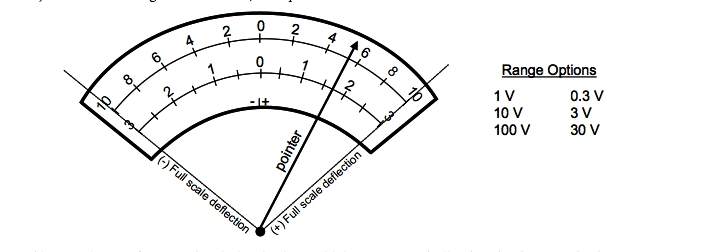# Reading an Analog Meter, given ranges

• Sarah Dabaja
It's a 0.3V scale, remember?Umm... If the range setting is 0.3V full scale, how close to full scale would 0.3V be and how close to full scale would 0.25V be?ADDENDUM TO POST #11:If the range setting is 0.3V full scale, how close to full scale would 0.3V be and how close to full scale would 0.25V be?0.3V would be at the very top of the scale, while 0.25V would be just below it. So they are both very close to full scale, but 0.3V is slightly closer.

## Homework Statement

1. Let’s say you know that the input signal will be about -0.25 V. What range should you select to give maximum deflection without going off scale? Draw a line, with a straight-edge, on the picture above, showing how the pointer would point. Below is the picture of the analog meter.

## The Attempt at a Solution

The range selected would be 10V, then divide 10V by 10 (the range) giving you 1V. After this, -.25V is divided by the 1V, giving you -.25V. I am not sure if doing it this way is correct?Sarah Dabaja said:

## Homework Statement

1. Let’s say you know that the input signal will be about -0.25 V. What range should you select to give maximum deflection without going off scale? Draw a line, with a straight-edge, on the picture above, showing how the pointer would point. Below is the picture of the analog meter.

## The Attempt at a Solution

The range selected would be 10V, then divide 10V by 10 (the range) giving you 1V. After this, -.25V is divided by the 1V, giving you -.25V. I am not sure if doing it this way is correct? View attachment 100459
Welcome to the PF.

Your solution drawing does not look correct. You see that the meter display can show either + or - values, right? And 0.25 is closest to (without going over) which of the available ranges?

berkeman said:
Welcome to the PF.

Your solution drawing does not look correct. You see that the meter display can show either + or - values, right? And 0.25 is closest to (without going over) which of the available ranges?
.25 would be closest to the 0.3 V range.

Sarah Dabaja said:
.25 would be closest to the 0.3 V range.
Correct! So if you set the meter to the +/- 0.3V full-scale range, where will the needle point when you put in the 0.25V test signal?berkeman said:
Correct! So if you set the meter to the +/- 0.3V full-scale range, where will the needle point when you put in the 0.25V test signal?Great! Would I have to do a calculation to figure out where the needle will point?

Sarah Dabaja said:
Great! Would I have to do a calculation to figure out where the needle will point?
Nope. Just draw where it would be on the meter. Remember, you are using the lower scale, with negative full scale as -0.30V and positive full scale as +0.30V. Where does the needle point to show -0.25V?

berkeman said:
Nope. Just draw where it would be on the meter. Remember, you are using the lower scale, with negative full scale as -0.30V and positive full scale as +0.30V. Where does the needle point to show -0.25V?
I would say -0.30V, but for deflection would it be the +0.30 V?

Sarah Dabaja said:
I would say -0.30V, but for deflection would it be the +0.30 V?
No. The needle will point on the meter face so that you will be able to read the voltage as -0.25V. So the needle can't deflect all the way to -0.30V, or you would mis-read the voltage.

Think of those scales as just number lines. If you have a number line from 0 to 10, and the value you want to indicate is 8, where would you point your finger along the number line?berkeman said:
No. The needle will point on the meter face so that you will be able to read the voltage as -0.25V. So the needle can't deflect all the way to -0.30V, or you would mis-read the voltage.

Think of those scales as just number lines. If you have a number line from 0 to 10, and the value you want to indicate is 8, where would you point your finger along the number line?You would point your finger to the line 8. So the needle will be pointed at -0.25V on the lower scale (somewhere in between the 0 and 0.5)?

•berkeman
Yes! Sorry it took me so long to respond.Umm... If the range setting is 0.3V full scale, how close to full scale would 0.3V be and how close to full scale would 0.25V be?